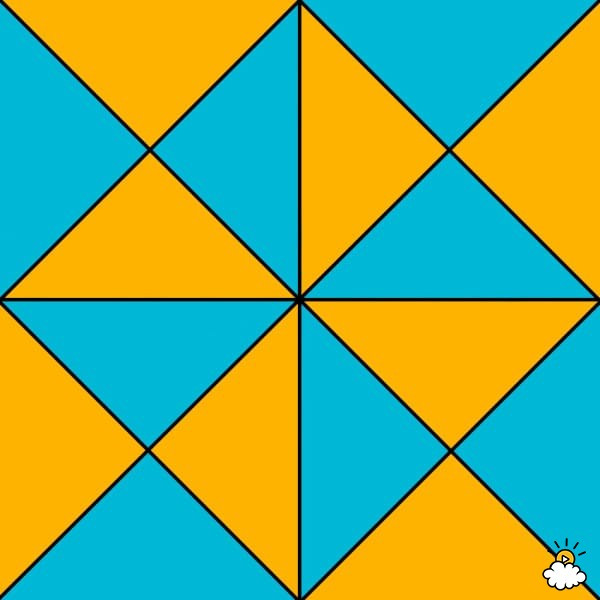Brainy Puzzles
Puzzle Solvers: Interesting brainy puzzles shared by fellow puzzlers
359 Members
Join this group to post and comment.Ankita Katdare • Dec 18, 2018

# How many triangles are there in this image? - Internet's puzzles

The Internet is full of puzzles and here is one. Can you count how many triangles are there in this image?

Fastest to count with explanation is the winner. ?Kaustubh Katdare • Dec 18, 2018

Is there any strategy to count? Let's see -

The biggest square has : - 8 triangles created by diagonals. Then, each of the smaller squares - add 8 new triangles. So total is 8 + 4x8 = 40 triangles.

Assuming I didn't miss any combination; my answer is 40 triangles. ?

Share this content on your social channels -